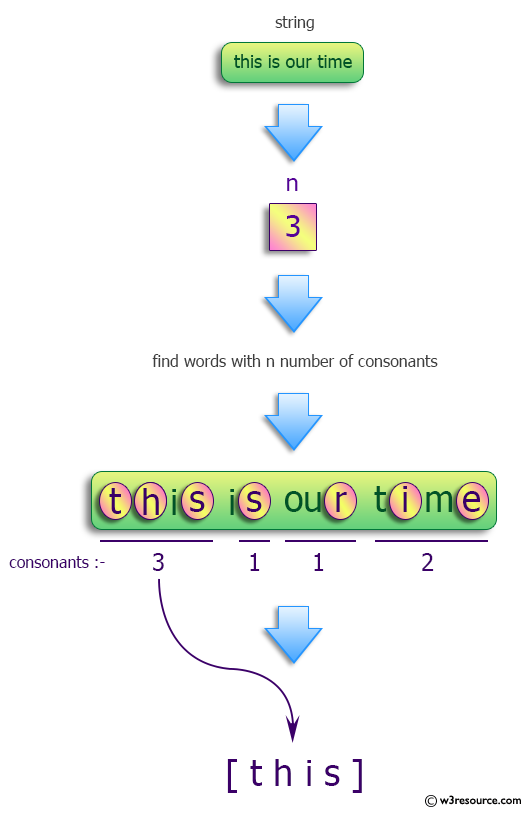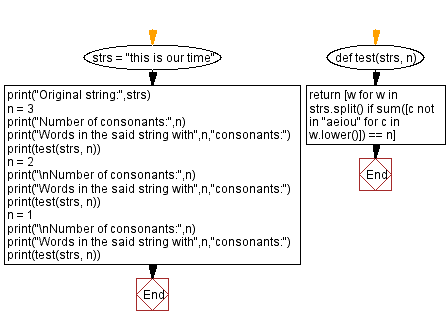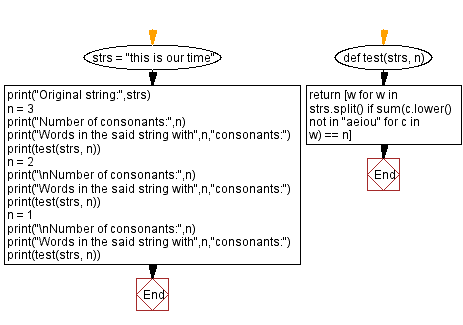﻿ Python: Find all words in a given string with n consonants - w3resource# Python: Find all words in a given string with n consonants

## Python Programming Puzzles: Exercise-43 with Solution

From vocabulary.com:
A consonant is a speech sound that is not a vowel. It also refers to letters of the alphabet that represent those sounds: Z, B, T, G, and H are all consonants. Consonants are all the non-vowel sounds, or their corresponding letters: A, E, I, O, U and sometimes Y are not consonants.

Write a Python program to find all words in a given string with n consonants.

```Input: this is our time
Output:
Number of consonants: 3
Words in the said string with 3 consonants:
['this']

Number of consonants: 2
Words in the said string with 2 consonants:
['time']

Number of consonants: 1
Words in the said string with 1 consonants:
['is', 'our']
```

Pictorial Presentation:Sample Solution-1:

Python Code:

``````#License: https://bit.ly/3oLErEI

def test(strs, n):
return [w for w in strs.split() if sum([c not in "aeiou" for c in w.lower()]) == n]
strs = "this is our time"
print("Original string:",strs)
n = 3
print("Number of consonants:",n)
print("Words in the said string with",n,"consonants:")
print(test(strs, n))
n = 2
print("\nNumber of consonants:",n)
print("Words in the said string with",n,"consonants:")
print(test(strs, n))
n = 1
print("\nNumber of consonants:",n)
print("Words in the said string with",n,"consonants:")
print(test(strs, n))
``````

Sample Output:

```Original string: this is our time
Number of consonants: 3
Words in the said string with 3 consonants:
['this']

Number of consonants: 2
Words in the said string with 2 consonants:
['time']

Number of consonants: 1
Words in the said string with 1 consonants:
['is', 'our']
```

Flowchart:## Visualize Python code execution:

The following tool visualize what the computer is doing step-by-step as it executes the said program:

Sample Solution-2:

Python Code:

``````def test(strs, n):
return [w for w in strs.split() if sum(c.lower() not in "aeiou" for c in w) == n]
strs = "this is our time"
print("Original string:",strs)
n = 3
print("Number of consonants:",n)
print("Words in the said string with",n,"consonants:")
print(test(strs, n))
n = 2
print("\nNumber of consonants:",n)
print("Words in the said string with",n,"consonants:")
print(test(strs, n))
n = 1
print("\nNumber of consonants:",n)
print("Words in the said string with",n,"consonants:")
print(test(strs, n))
``````

Sample Output:

```Original string: this is our time
Number of consonants: 3
Words in the said string with 3 consonants:
['this']

Number of consonants: 2
Words in the said string with 2 consonants:
['time']

Number of consonants: 1
Words in the said string with 1 consonants:
['is', 'our']
```

Flowchart:## Visualize Python code execution:

The following tool visualize what the computer is doing step-by-step as it executes the said program:

Python Code Editor :

Have another way to solve this solution? Contribute your code (and comments) through Disqus.

What is the difficulty level of this exercise?

Test your Programming skills with w3resource's quiz.

﻿

## Python: Tips of the Day

Clamps num within the inclusive range specified by the boundary values x and y:

Example:

```def tips_clamp_num(num,x,y):
return max(min(num, max(x, y)), min(x, y))
print(tips_clamp_num(2, 4, 6))
print(tips_clamp_num(1, -1, -6))
```

Output:

```4
-1
```